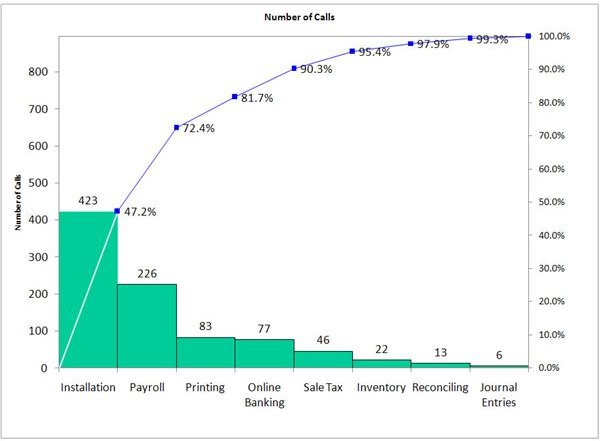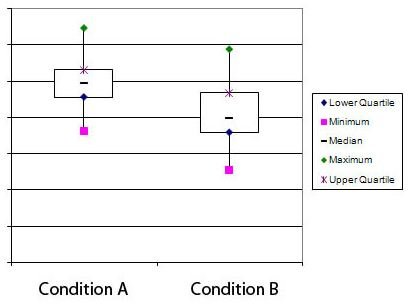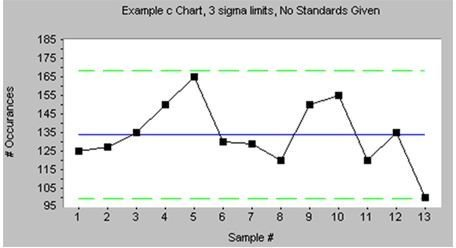# Guide to Six Sigma Data Analysis: 6 Tools - Histogram, Scatterplot, Boxplot, Pareto Chart, Time Series Plot, Control Chart

Page content

## Tools for Six Sigma Data Analysis

Histogram

A histogram is a common type of graph used to show the frequency of occurrence of different values. It is essentially a bar chart which is used to show the distribution of data gathered within a specific time period. The x-axis represents the values present in the data, while the y-axis (and thus the height of each bar) represents the frequency of occurence of that value or range of values.

The benefit of using a histogram is that it is simple to create and to understand, and most people in a business setting are accustomed to viewing such graphs. Use a histogram when you have numerical data and want to understand the data distribution, including its shape and central tendency.

Learn to create a histogram in Excel in Michele McDonough’s two-part series, Six Sigma Histograms in Microsoft Excel.

Scatterplot

A scatterplot or scatter diagram visually depicts the distribution of data when both the x and y variables are numerical. For instance, a scatterplot could show the number of births each year plotted against the number of storks spotted that year. With this type of graph you can easily see whether there is any possibility that the two variables are related. If not, the data points will be scattered randomly. If a strong linear relationship exists, the dots will be scattered as if drawn along an invisible line. If the relationship is weaker, the dots will be arranged more loosely but still show a tendency for the y variable to either increase or decrease as the x variable increases.

Use this type of graph when you have two numerical variables and are interested in the relationship between them. In most programs you can also add a line of best fit and determine if there is a statistically significant correlation.

Create a scatterplot in Excel using these instructions: Microsoft Excel 2007: Create a Scatter Plot.

Pareto ChartA Pareto chart may look at first glance like a histogram, but there are two key differences. The first is that the x variable is categorical rather than numerical. For instance, the x variable may be type of defect. The second difference is that the bars are arranged in decreasing order of frequency.

Use a Pareto chart when you are exploring the distribution of data across categories, particularly if you are trying to figure out how to focus your efforts. Analyzing the data with this tool lets you assess which categories are most frequent and whether a few categories represent a majority of the data.

Read more about Pareto charts in my article, The Pareto Principle and Its Application in Six Sigma.

Box-and-Whiskers PlotBox-and-whiskers plots, or boxplots for short, are helpful when you are interested in the details of the distribution of numerical data. They are especially useful for comparing numerical data across multiple groups or categories.

From a boxplot you can quickly get information about the mean or median of the data, the overall distribution and degree of variation, and the existence of outliers. You can also see how greatly distributions for different groups overlap. Boxplots are often generated as part of an analysis of variance (ANOVA) in programs such as Minitab.

Read more about boxplots in my article, Using Box-and-Whiskers Plots.

Time Series Plot

A time series plot is a graph that shows how your data changes over time. It is simpler than a control chart, and is useful if you want to get a quick look at the data to see if there are trends or obvious outliers.

Control ChartAnyone who has spent any time learning about Six Sigma is likely to be aware of control charts, as they are a hallmark of Six Sigma data analysis. A control chart is a special type of time series plot that incorporates statistical process control (SPC). Specifically, the upper and lower control limits are calculated and shown, to represent the range of values you could expect to have if your process is not affected by special cause. That is, it shows the amount of variation inherent in your process itself.

Control chart analysis reveals the presence of various types of special cause in the form of trends, outliers, and more. Use it for most DMAIC projects and any time you want to analyze your data to learn about variation and changes over time.